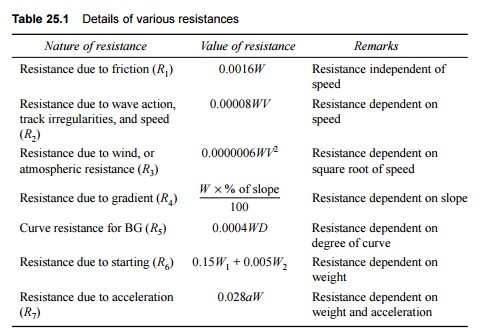Home | | Railways and Airports and Harbour Engineering | Train Resistance Due to Starting and Accelerating

# Train Resistance Due to Starting and Accelerating

Trains face these resistances at stations when they start, accelerate, and decelerate.

Various forces offer resistance to the movement of a train on the track. These resistances may be a result of the movement of the various parts of the locomotives as well as the friction between them, the irregularities in the track profile, or the atmospheric resistance to a train moving at great speed. The tractive power of a locomotive should be adequate enough to overcome these resistances and haul the train at a specified speed.

Resistance Due to Starting and Accelerating

Trains face these resistances at stations when they start, accelerate, and decelerate. The values of these resistances are as follows:

Resistance on starting, R6 = 0.15W1 + 0.005W2          (25.10)

Resistance due to acceleration, R7 = 0.028aW     (25.11)

where W1 is the weight of the locomotive in tonnes, W2 is the weight of the vehicles in tonnes, W is the total weight of the locomotive and vehicle in tonnes, i.e. W1 + W2, and a is the acceleration, which can be calculated by finding the increase in velocity per unit time, i.e., (V2 -        V1)/t, where V2 is the final velocity, V1 is the initial velocity, and t is the time taken.

Table 25.1 summarizes the various resistances faced by a train.

Table 25.1   Details of various resistancesStudy Material, Lecturing Notes, Assignment, Reference, Wiki description explanation, brief detail
Civil : Railway Airport Harbour Engineering : Railway Engineering : Train Resistance and Tractive Power : Train Resistance Due to Starting and Accelerating |

Related Topics